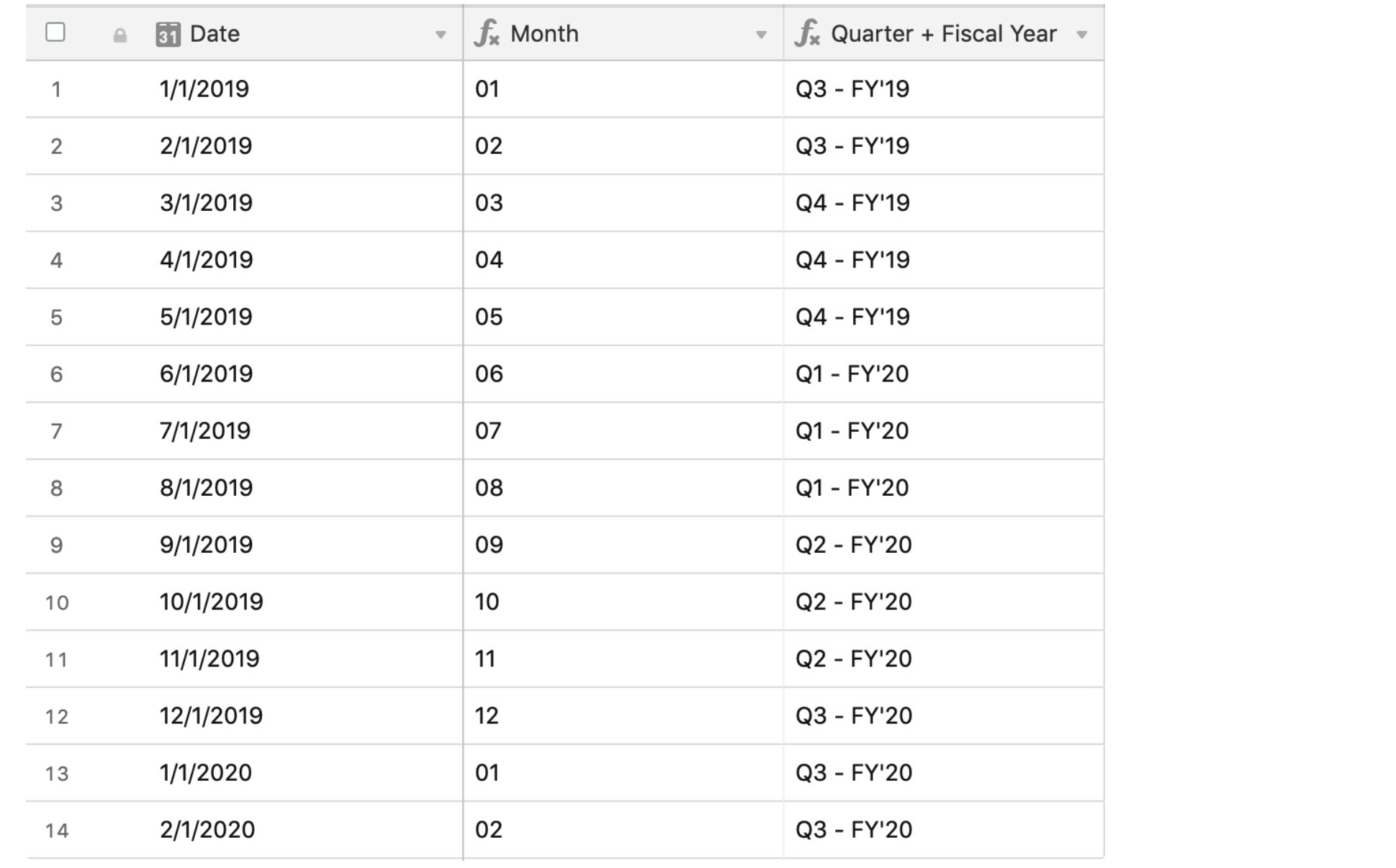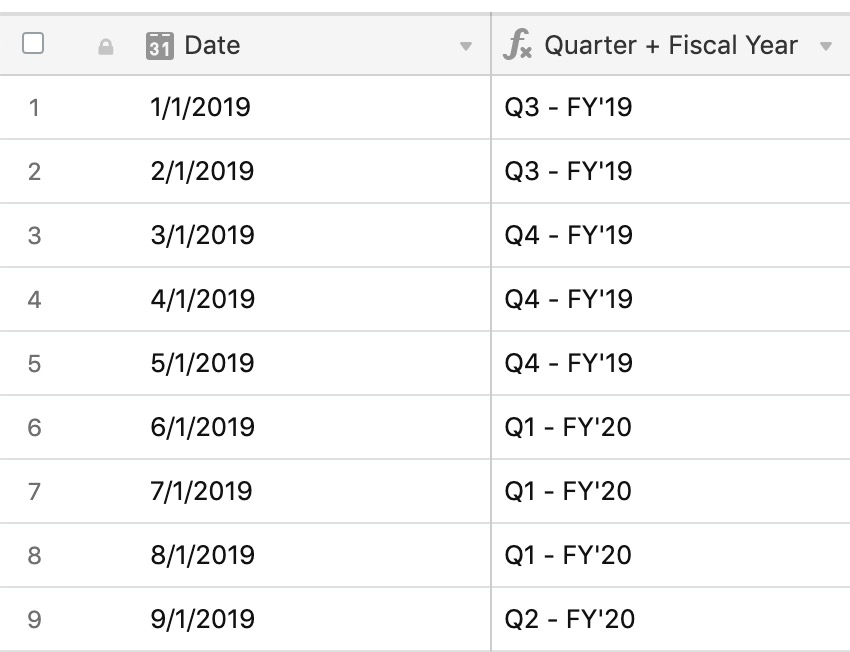• Account
• API
• Integrations
• Enterprise
• Resources
Displaying quarters in a fiscal year
• 06 Jun 2023
• Dark
Light

# Displaying quarters in a fiscal year

• Dark
Light

Article Summary

You can use a formula to display the quarter of a fiscal year based on the date in another field, even if your fiscal year is different from the calendar year.

As an example, let's say your fiscal year runs from June through May; that means your first quarter (Q1) of the year would start on June 1. You can build a formula to output the correct quarter and fiscal year based on an existing date field.

## Step 1

To start, one formula field will be used to output just the month (based on the date field) using the formula below:

``````IF(
{DATE},
0)
)``````

## Step 2

Once that's configured, a second formula will reference the Date and Month field, and generate the rest:

``````IF(
{DATE},
"FY'" & IF(
VALUE(DATETIME_FORMAT({Month}, 'M'))>=6,
DATETIME_FORMAT({Month}, 'YY'))
)``````

This will output and format the quarter and fiscal year of the record depending upon the information in the Date and Month fields:## Step 3 (optional)

If you'd prefer to not use two formula fields to accomplish this, you can combine the two previous formula fields into a single one. Here's how that formula would look altogether:

``````IF(
{Date},